6265.0 - Underemployed Workers, Australia, Sep 2009
Previous ISSUE Released at 11:30 AM (CANBERRA TIME) 23/02/2010
Page tools:Print AllRSSSearch this Product

TECHNICAL NOTE DATA QUALITY

INTRODUCTION

1 Estimates in this publication are based on information obtained from occupants of a sample of dwellings, and are subject to sampling variability. That is, they may differ from those estimates that would have been produced if all dwellings had been included in the survey. One measure of the likely difference is given by the standard error (SE), which indicates the extent to which an estimate might have varied by chance because only a sample of dwellings was included. There are about two chances in three (67%) that a sample estimate will differ by less than one SE from the number that would have been obtained if all dwellings had been included, and about 19 chances in 20 (95%) that the difference will be less than two SEs. Another measure of the likely difference is the relative standard error (RSE), which is obtained by expressing the SE as a percentage of the estimate.

2 Due to space limitations, it is impractical to print the SE of each estimate in the publication. Instead, a table of SEs is provided to determine the SE for an estimate from the size of that estimate (see table T1). The SE table is derived from a mathematical model, referred to as the 'SE model', which is created using data from a number of past Labour Force Surveys. It should be noted that the SE model only gives an approximate value for the SE for any particular estimate, since there is some minor variation between SEs for different estimates of the same size.

3 The LFS sample size in September 2009 was approximately 9% higher than the sample size in 2008. This is due to re-instated sample that was cut from Labour Force Survey (LFS) and supplementary surveys from July 2008. In combination, the sample re-instatement is expected to decrease the standard errors for estimates from the supplementary surveys by approximately 4% at the broad aggregate level, relative to estimates from 2008 (standard errors will vary at lower aggregate levels). Detailed information about the sample reduction/re-instatement is provided in Information Paper: Labour Force Survey Sample Design, Nov 2007 (Third edition) (cat. no. 6269.0).

CALCULATION OF STANDARD ERROR

4 An example of the calculation and the use of SEs in relation to estimates of persons is as follows. Table 4 shows the estimated number of female underemployed part-time workers was 452,100. Since this estimate is between 300,000 and 500,000, table T1 shows that the SE for Australia will lie between 7,300 and 9,200 and can be approximated by interpolation using the following general formula: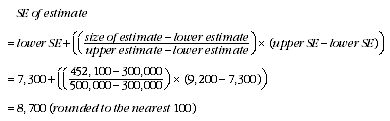5 Therefore, there are about two chances in three that the value that would have been produced if all dwellings had been included in the survey will fall within the range 443,400 to 460,800 and about 19 chances in 20 that the value will fall within the range 434,700 to 469,500. This example is illustrated in the following diagram.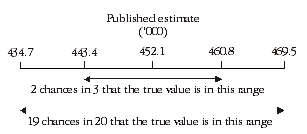6 In general, the size of the SE increases as the size of the estimate increases. Conversely, the RSE decreases as the size of the estimate increases. Very small estimates are thus subject to such high RSEs that their value for most practical purposes is unreliable. In the tables in this publication, only estimates with RSEs of 25% or less are considered reliable for most purposes. Estimates with RSEs greater than 25% but less than or equal to 50% are preceded by an asterisk (e.g.*3.2) to indicate they are subject to high SEs and should be used with caution. Estimates with RSEs of greater than 50%, preceded by a double asterisk (e.g.**0.3), are considered too unreliable for general use and should only be used to aggregate with other estimates to provide derived estimates with RSEs of less than 25%.

MEANS AND MEDIANS

7 The RSEs of estimates of mean duration of insufficient work, median duration of insufficient work and mean preferred number of extra hours are obtained by first finding the RSE of the estimate of the total number of persons contributing to the mean or median (see table T1) and then multiplying the resulting number by the following factors:
• mean duration of insufficient work: 1.6
• median duration of insufficient work: 2.5
• mean preferred number of extra hours: 0.7

8 The following is an example of the calculation of SEs where the use of a factor is required. Table 4 shows that the estimated number of male underemployed part-time workers was 283,800 with a median duration of insufficient work of 26 weeks. The SE of 283,800 can be calculated from table T1 (by interpolation) as 7,100. To convert this to an RSE we express the SE as a percentage of the estimate or 7,100/283,800 = 2.5%.

9 The RSE of the estimate of median duration of insufficient work is calculated by multiplying this number (2.5%) by the appropriate factor shown in paragraph 7 (in this case 2.5): 2.5 x 2.5 = 6.3%. The SE of this estimate of median duration of insufficient work is therefore 6.3% of 26, i.e. about 2 (rounded to the nearest whole week). Therefore, there are two chances in three that the median duration of insufficient work for males that would have been obtained if all dwellings had been included in the survey would have been within the range 24-28 weeks, and about 19 chances in 20 that it would have been within the range 22-30 weeks.

PROPORTIONS AND PERCENTAGES

10 Proportions and percentages formed from the ratio of two estimates are also subject to sampling errors. The size of the error depends on the accuracy of both the numerator and the denominator. A formula to approximate the RSE of a proportion is given below. This formula is only valid when x is a subset of y.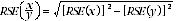11 Considering the example from paragraph 3, of the 452,100 female underemployed part-time workers, 153,600 or 34.0% had insufficient work for 52 weeks and over. The SE of 153,600 may be calculated by interpolation as 5,500. To convert this to an RSE we express the SE as a percentage of the estimate, or 5,500/153,600 = 3.6%. The SE for 452,100 was calculated previously as 8,700, which converted to an RSE is 8,700/452,100 = 1.9%. Applying the above formula, the RSE of the proportion is: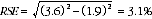12 Therefore, the SE for the proportion of females who have a current period of insufficient work of 52 weeks or more is 1.1 percentage points (=(34.0/100)x3.1). Therefore, there are about two chances in three that the proportion of females who have a current period of insufficient work of 52 weeks or more was between 32.9% and 35.1% and 19 chances in 20 that the proportion is within the range 31.8% to 36.2%.

DIFFERENCES

13 Published estimates may also be used to calculate the difference between two survey estimates (of numbers or percentages). Such an estimate is subject to sampling error. The sampling error of the difference between two estimates depends on their SEs and the relationship (correlation) between them. An approximate SE of the difference between two estimates (x-y) may be calculated by the following formula:

14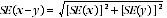15 While this formula will only be exact for differences between separate and uncorrelated characteristics or subpopulations, it is expected to provide a good approximation for all differences likely to be of interest in this publication.

STANDARD ERRORS

 T1 STANDARD ERRORS OF ESTIMATESAUST.NSW Vic. Qld. SA WA Tas. NT ACT SE RSE Size of Estimate (persons) no. no. no. no. no. no. no. no. no. % 100 320 310 240 190 240 120 80 110 120 120.0 200 440 420 350 260 320 170 120 180 210 105.0 300 520 490 430 310 380 210 150 230 290 96.7 500 640 600 550 380 460 260 200 300 420 84.0 700 730 680 640 430 530 300 230 330 530 75.7 1,000 840 780 750 500 600 340 270 370 670 67.0 1,500 990 910 890 580 710 400 310 400 860 57.3 2,000 1 110 1 020 1 000 650 790 440 340 430 1 010 50.5 2,500 1 200 1 100 1 100 700 850 450 350 450 1 150 46.0 3,000 1 300 1 200 1 150 750 900 500 400 500 1 250 41.7 3,500 1 400 1 250 1 250 800 950 500 400 500 1 350 38.6 4,000 1 450 1 350 1 300 850 1 000 550 450 500 1 450 36.3 5,000 1 600 1 450 1 400 900 1 100 600 500 550 1 650 33.0 7,000 1 800 1 650 1 600 1 000 1 250 650 550 650 1 900 27.1 10,000 2 050 1 850 1 750 1 150 1 400 750 750 750 2 200 22.0 15,000 2 400 2 150 2 000 1 300 1 650 950 1 000 950 2 550 17.0 20,000 2 650 2 400 2 150 1 450 1 800 1 100 1 300 1 100 2 850 14.3 30,000 3 100 2 800 2 500 1 750 2 100 1 350 1 800 1 350 3 200 10.7 40,000 3 400 3 100 2 750 2 000 2 300 1 650 2 250 1 650 3 500 8.8 50,000 3 700 3 350 3 050 2 200 2 550 1 850 2 700 1 850 3 750 7.5 100,000 4 700 4 400 4 150 3 300 3 750 2 650 4 650 2 450 4 750 4.8 150,000 5 550 5 350 5 100 4 250 4 950 3 150 6 400 2 750 5 500 3.7 200,000 6 350 6 250 5 950 5 000 5 950 3 550 8 000 2 950 6 150 3.1 300,000 8 000 7 950 7 550 6 150 7 450 4 050 10 950 3 100 7 300 2.4 500,000 11 200 11 050 10 200 7 700 9 500 4 700 . . 3 100 9 200 1.8 1,000,000 16 650 16 850 14 550 9 800 12 100 5 350 . . . . 12 950 1.3 2,000,000 22 450 24 900 19 600 11 650 14 000 . . . . . . 18 800 0.9 5,000,000 28 600 39 800 26 400 13 150 14 600 . . . . . . 31 400 0.6 10,000,000 30 650 54 900 30 900 . . . . . . . . . . 41 900 0.4 15,000,000 . . . . . . . . . . . . . . . . 47 250 0.3 . . not applicable